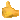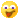# Latest posts

8 + 8 = ?

20 + 20 = ?

100 + 100 = ?

## school

?did we get any homework because I was of school## how to make 30

5+5+5+5+5+5=30                                                                                                                          2+2+2+2+2+2+2+1=30                                                                                                                 100-70=30                                                                                                                                    20+20-10=30                                                                                                                               10+10+10=30that was hard

## Hi 2RS

Hi Miss Schofield and 2RS, I hope you have a good weekend see you next week.